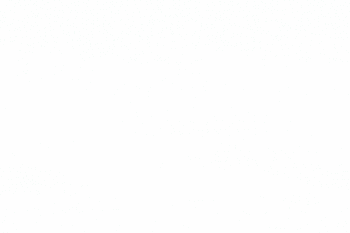Limiting reactant and excess reactantLimiting Reagent

↪ The  reactant or the chemical reagent which is present in lesser amount than required by balanced chemical equation.
↪ The reagent that gets finished at first during reaction is called limiting reagent.
↪ Limiting reagent is important to determine the amount of product formed during the chemical reaction.

Excess Reagent

↪ The reactant which is present in excess amount as required by the balanced chemical equation.
↪ The reagent which is left over at the end of any chemical reaction is called  excess reagent.

To illustrate the concept of limiting reagent, consider reaction between zinc metal and sulphuric acidMass relationship of balanced equation shows that 65 g of zinc reacts completely with 98 g of H2SO4 .

If zinc and H2SO4 are mixed in the ratio of 65 : 98 by mass, there must be complete reaction i.e. at the end, non of the reactant remain unreacted.

In this reaction ZnSO4 and H2 are obtained in the ratio of 161 : 2 by mass.

In an experiment, if 6.5 g of zinc is mixed with 9 g of H2SO4  get exhausted earlier leaving a fraction by mass of zinc unreacted.

From mass-mass relationship of above balanced equation, we know that 9 g of H2SO4 can react only with 5.97 g i.e.i.e. a fraction by mass of zinc (6.5 – 5.97 = 0.53 g) remain unreacted.

Hence, zinc is excess reactant while H2SO4 is the limiting reactant in this reaction.

Now, the mass of ZnSO4 and H2 produced depend upon the mass of H2SO4 reacted.